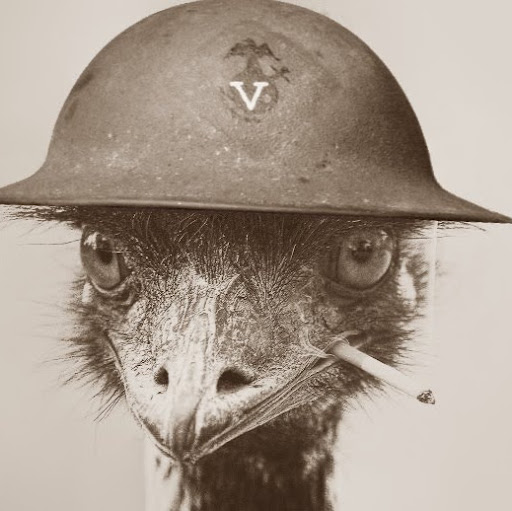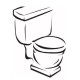# Visualizing Quaternions with Unity

How do you model the position and orientation of an airplane?

Position is easy, just represent it with a point in 3D space. But how do you specify its orientation — which direction it’s pointing?

At first glance, it seems a vector will do. After all, a vector points in some direction, right? If the plane is pointing east, represent its orientation by a unit vector pointing east.Unfortunately, we quickly run into trouble when we try to roll. If we’re facing east, and we roll 90 degrees, we’re still facing east. Clearly we’re missing something.

### Euler Angles

When real pilots talk about their orientation, they talk about roll, yaw, pitch. Pitch is going up or down, yaw is going left or right, roll is, well, roll.Any change in orientation can be described by some combination of roll, yaw, pitch. This is the basis for Euler Angles. We use three angles to represent the airplane’s orientation.

This is all fine and dandy if we want to represent the orientation of a static object in space. But when we try to adjust our orientation, we start to run into problems.

You’re thinking, this should be simple! When we turn left or right, we just increment the yaw variable, right? Yes, it seems to work, at least initially. You can turn left and right, up and down, and roll around.

Implement it in Unity and play around a bit, however, and you begin to notice that things don’t quite behave the way you expect.

In this animation, I’m holding down the right button:The plane does rotate to the right, but it’s not rotating relative to itself. Instead it’s rotating around some invisible y-axis. If it was rotating relative to itself, the green arrow shouldn’t be moving.

The problem becomes more and more severe when the pitch of the plane becomes higher and higher. The worst case is when the airplane is pointing straight up: then roll and yaw become the same thing! This is called gimbal lock: we have lost a degree of freedom and we can only rotate in 2 dimensions! Definitely not something desirable if we’re controlling a plane or spaceship.

It turns out that no matter what we do, we will suffer from some form of gimbal lock. As long as we use Euler Angles, there is one direction where if we turn too far, everything starts to screw up.

### Practical Introduction to Quaternions

All is not lost, however. There is a way to represent orientation that represents all axes equally and does not suffer from gimbal lock. This mythical structure is called the quaternion. Unlike Euler Angles which describe your orientation relative to a fixed set of axes, quaternions do not rely on any fixed axis.

The drawback is that quaternions are unintuitive to understand for humans. There is no way to “look” at a quaternion and be able to visualize what rotation it represents. Fortunately for us, it’s not that difficult to make use of quaternions, even if we can’t visualize quaternions.

There is a lot of theory behind how quaternions work, but in this article, I will gloss over the theory and give a quick primer to quaternions, just the most common facts you need to use them. At the same time, I will implement the operations I describe in C#, so I can integrate them with Unity. If you don’t know C#, you can freely ignore the code.

### Definition

A quaternion is an ordered pair of 4 real numbers (w,x,y,z). We write this as$w+xi+yj+zk$

The letters i,j,k are not variables. Rather, they are independent axes. If you like, you can think of the quaternions as a 4 dimensional vector space.

The defining property of quaternions is:$i^2 = j^2 = k^2 = ijk = -1$

Play around with it a bit and you can derive 6 more identites:$ij = k$$jk = i$$ki = j$$ji = -k$$kj = -i$$ik = -j$

If you’ve worked with complex numbers, this should seem familiar. Instead of 2 parts of a complex number (the real and imaginary parts), we have 4 parts for a quaternion.

The similarity doesn’t end here. Multiplying complex numbers represents a rotation in 2 dimensions. Similarly, multiplying by a quaternion represents a rotation in 3D.

One curious thing to note: we have$ij=k$ and$ji=-k$. We switched around the terms and the product changed. This means that multiplying quaternions is kind of like multiplying matrices — the order matters. So multiplication is not commutative.

Here’s a framework for a quaternion in C#:

public class Quat{
// Represents w + xi + yj + zk
public float w, x, y, z;
public Quat(float w, float x, float y, float z){
this.w = w;
this.x = x;
this.y = y;
this.z = z;
}
}


### Normalizing Quaternions

The norm of a quaternion is$N(\mathbf{q}) = \sqrt{w^2+x^2+y^2+z^2}$

When we use quaternions to represent rotations, we typically want unit quaternions: quaternions with norm 1. This is straightforward: to normalize a quaternion, divide each component by the norm.

In C#:

public float Norm(){
return Mathf.Sqrt (w * w + x * x + y * y + z * z);
}

public Quat Normalize(){
float m = Norm ();
return new Quat (w / m, x / m, y / m, z / m);
}


### Multiplying Quaternions

Multiplying is simple, just a little tedious. If we have two quaternions:$(w_1 + x_1i + y_1j + z_1k) (w_2+x_2i+y_2j+z_2k)$

Then their product is this ugly mess:$\begin{array}{l} w_1w_2-x_1x_2-y_1y_2-z_1z_2 \\ + (w_1x_2+x_1w_2+y_1z_2-z_1y_2)i \\ + (w_1y_2+y_1w_2-x_1z_2+z_1x_2) j \\ + (w_1z_2+z_1w_2+x_1y_2-y_1x_2) k \end{array}$

In C#:

// Returns a*b
public static Quat Multiply(Quat a, Quat b){
float w = a.w * b.w - a.x * b.x - a.y * b.y - a.z * b.z;
float x = a.w * b.x + a.x * b.w + a.y * b.z - a.z * b.y;
float y = a.w * b.y + a.y * b.w - a.x * b.z + a.z * b.x;
float z = a.w * b.z + a.z * b.w + a.x * b.y - a.y * b.x;
return new Quat (w,x,y,z).Normalize();
}


Since multiplication is not commutative, I made this function static to avoid confusing left and right multiplication. Also, I normalize the product so that floating point errors don’t accumulate.

### Constructing Rotation Quaternions

Every rotation operation can be written as a rotation of some angle,$\theta$, around some vector$(u_x, u_y, u_z)$:The following formula gives a quaternion that represents this rotation:$\mathbf{q} = \cos \frac{\theta}{2} + (u_x i + u_y j + u_z k) \sin \frac{\theta}{2}$

For our purposes,$\theta$ is a very small number, say 0.01, and we use one of the three basis vectors to rotate around. For example, if we are rotating around (1,0,0) then our quaternion is$\cos \frac{0.01}{2} + \sin \frac{0.01}{2}i$

That’s it: given any quaternion, multiplying on the left by our quaternion rotates it slightly around the x axis.

In C#, our code might look like this:

Quat qx = new Quat (Mathf.Cos (0.01 / 2), 0, 0, Mathf.Sin (0.01 / 2));
Quat qy = new Quat (Mathf.Cos (0.01 / 2), 0, Mathf.Sin (0.01 / 2), 0);
Quat qz = new Quat (Mathf.Cos (0.01 / 2), Mathf.Sin (0.01 / 2), 0, 0);


### Putting it together

That’s all we need to do interesting things with quaternions. Let’s combine everything we have. Here’s our quaternion class thus far:

public class Quat{
// Represents w + xi + yj + zk
public float w, x, y, z;
public Quat(float w, float x, float y, float z){
this.w = w;
this.x = x;
this.y = y;
this.z = z;
}

public float Norm(){
return Mathf.Sqrt (w * w + x * x + y * y + z * z);
}

public Quat Normalize(){
float m = Norm ();
return new Quat (w / m, x / m, y / m, z / m);
}

// Returns a*b
public static Quat Multiply(Quat a, Quat b){
float w = a.w * b.w - a.x * b.x - a.y * b.y - a.z * b.z;
float x = a.w * b.x + a.x * b.w + a.y * b.z - a.z * b.y;
float y = a.w * b.y + a.y * b.w - a.x * b.z + a.z * b.x;
float z = a.w * b.z + a.z * b.w + a.x * b.y - a.y * b.x;
return new Quat (w,x,y,z).Normalize();
}

public Quaternion ToUnityQuaternion(){
return new Quaternion (w, x, y, z);
}
}


Now we just need to read the input, perform our calculations, and output the rotation quaternion to Unity:

public class Airplane : MonoBehaviour {
public GameObject airplane;
public Quat quat = new Quat (0, 0, 0, -1);
public float speed = 0.01f;

void FixedUpdate(){
float inputX = Input.GetAxis("UpDown");
float inputY = Input.GetAxis("LeftRight");
float inputZ = Input.GetAxis("Roll");

Quat qx = new Quat (Mathf.Cos (speed * inputX / 2), 0, 0, Mathf.Sin (speed * inputX / 2));
Quat qy = new Quat (Mathf.Cos (speed * inputY / 2), 0, Mathf.Sin (speed * inputY / 2), 0);
Quat qz = new Quat (Mathf.Cos (speed * inputZ / 2), Mathf.Sin (speed * inputZ / 2), 0, 0);

quat = Quat.Multiply (qx, quat);
quat = Quat.Multiply (qy, quat);
quat = Quat.Multiply (qz, quat);

airplane.transform.rotation = quat.ToUnityQuaternion ();
}
}


In Unity, the input is not given to us as a single true/false value, but a float between -1 and 1. So holding right increases the LeftRight input gradually until it reaches 1, avoiding a sudden jump in movement.

What’s ToUnityQuaternion? Well, it turns out that Unity already has a Quaternion class that does everything here and much more, so all this could have literally been implemented in one line if we wanted.

Anyways, let’s see the result.As you can see, holding right turns the plane relative to itself now, and the green arrow stays still. Hooray!

## 10 thoughts on “Visualizing Quaternions with Unity”

1.Impressed reader says:

You are awesome!

Like

2.Anonymous says:

Excellent explanation

Like

3.Alexander Antonov (sambroadleaf) says:

Yeah apart from euler example plane where you simply forgot to use local coordinates.

Like

1.Alexander Antonov (sambroadleaf) says:

Gimbal lock is a real thing no doubt but your example is just wrong.

Like

2.luckytoilet says:

What’s the error? Gimbal lock is when rotating the object in a certain way makes it so now it only has 2 degrees of freedom, instead of 3.

Like

1.Ken Grimm says:

He’s saying that your example is not really a problem if you rotate around your local axis and not world. Using eulerAngles this does work.
https://docs.unity3d.com/ScriptReference/Transform.Rotate.html
Using relativeTo should fix that example.

Like

2.Ken Grimm says:

And btw, awesome write-up!

Like

4.Lucas says:

How could this be solved in one line of code ?

Like

5.Kambot says:

Are you sure you got this function right?
public Quaternion ToUnityQuaternion(){
return new Quaternion (w, x, y, z);
}
Unity defines the Quaternion elements in a different order:
public Quaternion(float x, float y, float z, float w);

This little problem would explain why you had to stretch the maths a bit to make the example work in the final part of the code.

Like